Next: The Sagnac experiment Up: Criticism of the relativistic experiments Previous: Aberration, the Fizeau experiment   Contents

## The Ritz hypothesis

For the sake of fairness, we note that even the Ritz ballistic hypothesis (in essence, it is the classical law of addition of velocities for corpuscles) could not so easily be disproved at the beginning of 20th century. We shall present briefly the derivation from  and make some comments. The time for a signal to arrive from a satellite of a central star at distance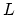is, upon entering the shadow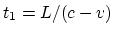, and upon exiting from the shadow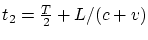, where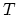is the orbit period. Allowing for a noticeable effect, the binary system will be seen as threefold when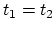, for which we obtain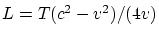. For the diameter of orbit we have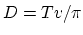. Ifis the observation angle, then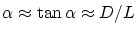, and, since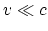, we have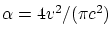. The real velocities of observed satellites are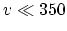km/s. As a result, for observation of a similar effect we must have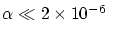radians (which is beyond the accuracy of modern telescopes).

Of course, this conclusion is rather rough. In the expression for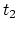instead of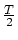one must write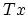, whereis the fraction of a period, when the satellite is in shadow; generally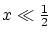, which increases the limiting accuracy of. Besides, very short time intervals can be recorded now by means of photography (if the exposure allows this), i.e. one may write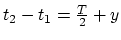, where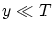, which ever more increases the limiting accuracy.

However, some remarks can be made for justification as well. Namely:
(1) The study of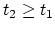is non-productive, since all observed eclipses will be periodic, and we can not verify in any way, whether we really observe a threefold (or fourfold, etc.) system, or this is only a semblance.
(2) During the orbital motion of a satellite the time of signal arrival to the observation point changes smoothly (the real object - a satellite - does not coincide with its visible image), which distorts the determination of a real orbital motion and quantity.
(3) Since the light passes through the inhomogeneous medium (the atmosphere, as well as the near-Earth space), the phenomena of scintillation and dispersion can take place. In order to lower their negative effect, the full (rather than partial) eclipses should be observed and, preferably, from the Earth artificial satellites.
(4) Because only the projection of the orbital plane will be accessible for us, we cannot, in the general case, estimate the valueof the section of shadow (Fig. 3.3).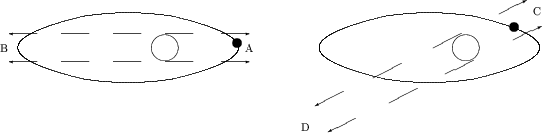The time of motion in a shadow will be different depending on the direction to the observer (to the Earth). Hence, the objects with symmetric orientation are required, and the accuracy of determination of "arms" of the orbit projection and of the size of both bodies imposes limitations on the (calculated) accuracy of determination of signals arrival times.
(5) We have already mentioned above, that the abstract speed of light does not exist, but particular values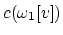and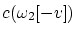will be observed. Therefore, the accuracy of determination of frequencies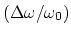imposes limitations on the theoretically calculated accuracy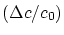and, accordingly, on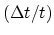.

The most important comment is as follows:
(6) The light of some frequency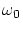is emitted, not by the object as a whole moving at velocity, but rather by the particles moving chaotically within the object with thermal velocities. Therefore, it is impossible to determine the delay of calculated time depending on the velocity of the object as a whole by using any characteristic (in microscales) frequencies (radiation lines). Only if the graph of satellite spectral intensity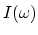possesses some particular characteristic form (for example, having a maximum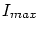at frequency), and ifdiffers identifiable from the graph of star spectral intensity (for example, in shape), can the observation of changes in spectral intensity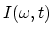at the variable frequency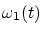prove or disprove the Ritz ballistic hypothesis.

As far as the author knows, no such detailed analysis of the astronomical data was carried out. It should be further mentioned that the Ritz hypothesis predicts for binary systems not only a phase modulation of the signal received, but an amplitude one as well (as the result of the varying speed of light propagation, in a fixed space point there occur a pulsation of an intensity due to superposition of light which was emitted at different time instants). As this takes place, the ralative intensity of pulsation increases with the distance to the binary system. The frequency of pulsations also increases (to some limits). Some authors  believe that the "existence" of quasars and pulsars is one of proofs of the Ritz hypothesis. Really, the smallness of their pulsation period (sometimes less than one second) testifies to the compactness of these objects, but the emitted radiant power (taking into account their remoteness) testifies against the first assumption. And either we must thoroughly test the Ritz hypothesis, or it remains to believe in modern fantastical (non-verifiable) versions. And complications with the processing of radar observations of the Venus compel to meditate on the possibility for the inertial properties of light to exist. However, the defence or development of Ritz's hypothesis is not a subject of this work.Next: The Sagnac experiment Up: Criticism of the relativistic experiments Previous: Aberration, the Fizeau experiment   Contents
Sergey N. Arteha